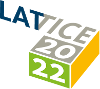#The 39th International Symposium on Lattice Field Theory (Lattice 2022)

Aug 8 – 13, 2022
Hörsaalzentrum Poppelsdorf
Europe/Berlin timezone

## Tuning of QCD+QED simulations with C$^\star$ boundary conditions

Aug 9, 2022, 8:00 PM
1h
Poster Presentation Hadron Spectroscopy and Interactions

### Speaker

Alessandro Cotellucci (Humboldt University Berlin)

### Description

We give an update on the ongoing effort of the RC$^\star$ collaboration to generate fully dynamical QCD+QED ensembles with C$^\star$ boundary conditions using the openQ$^\star$D code. The simulations were tuned to the U-symmetric point ($m_d=m_s$) with pions at $m_{\pi^{\pm}} \approx 400$ MeV. The splitting of the light mesons is used as one of three tuning observables and fixed to $m_{K^0}-m_{K^{\pm}} \approx 5$ MeV and $m_{K^0}-m_{K^{\pm}} \approx 25$ MeV on ensembles with renormalized electromagnetic coupling $\alpha_R \approx \alpha_{\text{phys.}}$ and $\alpha_R \approx 5.5 \alpha_{\text{phys.}}$ respectively. The tuning of the three independent quark masses to the desired line of constant physics is particularly challenging. In this poster we will define the chosen hadronic renormalization scheme, and we will present a tuning strategy based on a combination of mass reweighting and linear interpolation to explore the parameter space. We will comment on finite-volume effects comparing meson masses on two different volumes with $m_{\pi^\pm}L \approx 3.2$ and $m_{\pi^\pm}L \approx 5.1$. We will also provide some technical details on our updated strategy to calculate the sign of the fermionic Pfaffian, which arises in presence of C$^\star$ boundary conditions in place of the standard fermionic determinant. An overview of the QCD+QED configurations generated by the RC$^\star$ collaboration will be given in the companion talk presented by J. Lücke.

### Primary author

Alessandro Cotellucci (Humboldt University Berlin)

### Co-authors

Agostino Patella (Humboldt University Berlin) Anian Altherr (ETH Zürich) Isabel Campos Plasencia (Consejo Superior de Investigaciones Cientificas (CSIC) (ES)) Javad Komijani (ETH Zurich) Jens Lücke (Humboldt University Berlin) Joao C. Pinto Barros (ETH Zürich) Lucius Bushnaq Madeleine Dale Marco Catillo Marina Krstic Marinkovic (ETH Zurich) Nazario Tantalo (University and INFN of Rome Tor Vergata) Paola Tavella (ETH) Patrick Fritzsch (Trinity College Dublin) Roman Gruber (ETH Zürich)